Next: Synthetic data results Up: Robust inversion using the Previous: Implementation of a nonlinear

# Application of the Huber norm: velocity estimation with noisy data

In this section, the proposed algorithm is used on a geophysical inverse problem which is velocity estimation with noisy data. Some possible applications of a robust solver are, for example, tomography Bube and Langan (1997) and deconvolution of noisy data Chapman and Barrodale (1983). My goal is to demonstrate that the Huber function with the L-BFGS method gives a robust estimate of the model parameters when outliers are present in the data. The velocity estimation problem with the Huber norm has potential applications when multiples need to be separated from the signal in the velocity domain Kostov and Nichols (1995); Lumley et al. (1995). More conventional multiple attenuation techniques using the parabolic radon transform Herrmann et al. (2000); Kabir and Marfurt (1999) can also benefit from using the Huber norm.

The velocity domain'' representation of seismic data using the hyperbolic radon transform (HRT) is an alternative to the standard common midpoint (CMP) gather. Transformation of CMP data into the velocity domain (producing a velocity model or panel of the data) exhibits clearly the moveout inherent in the data and therefore, forms a convenient basis for velocity analysis as a linear inverse problem.

Thorson and Claerbout (1985) were the first to define the forward and adjoint operators of the HRT, formulating it as an inverse problem in which the velocity domain is the unknown space. In their approach the forward operator L maps the model space (velocity domain) into the data space (CMP gathers). This transformation is a superposition of hyperbolas in the data space. The adjoint operator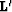, the HRT, maps the data space into the model space. This transformation is a summation over hyperbolic trajectories in the data space (related to the velocity stack as defined by Taner and Koehler (1969)). With d(t,x) being a CMP gather and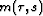the corresponding velocity model, the forward operation is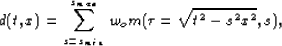(4)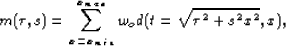(5)
where x is the offset (xmin and xmax being the offset range), s the slowness (smin and smax being the range of slownesses investigated),the two-way zero offset travel time, and wo a weighting function that compensates to some extent for geometrical spreading and other effects Claerbout and Black (1997).

Having defined the forward operator L and its adjoint, the inverse problem can be posed. Inverse theory helps us to find a velocity panel which synthesizes a given CMP gather via the operator L. In equations, given data d (CMP gather), we want to solve for the model m (velocity panel)(6)
which leads in a least-squares sense to the linear system (normal equations'')(7)
This system is easy to solve if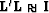, i.e., if L is close to unitary. Unfortunately, L is far from an unitary operator Kabir and Marfurt (1999); Sacchi and Ulrych (1995). In addition, the number of equations and unknowns may be large, making an iterative data-fitting approach reasonable.

Consequently, with E being a misfit measurement function, our goal is to iteratively calculate the modelthat minimizes the misfit function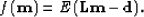(8)
One possibility for E is thenorm (least-squares inversion). The misfit function is then usually minimized with conjugate-gradient. Another possible approach is of course by taking the Huber norm along with the L-BFGS method introduced in the preceding section. The two norms are compared in the next section for velocity estimation problems. The results show that the Huber norm gives the expected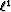behavior when outliers (non-gaussian noise) are present in the data.